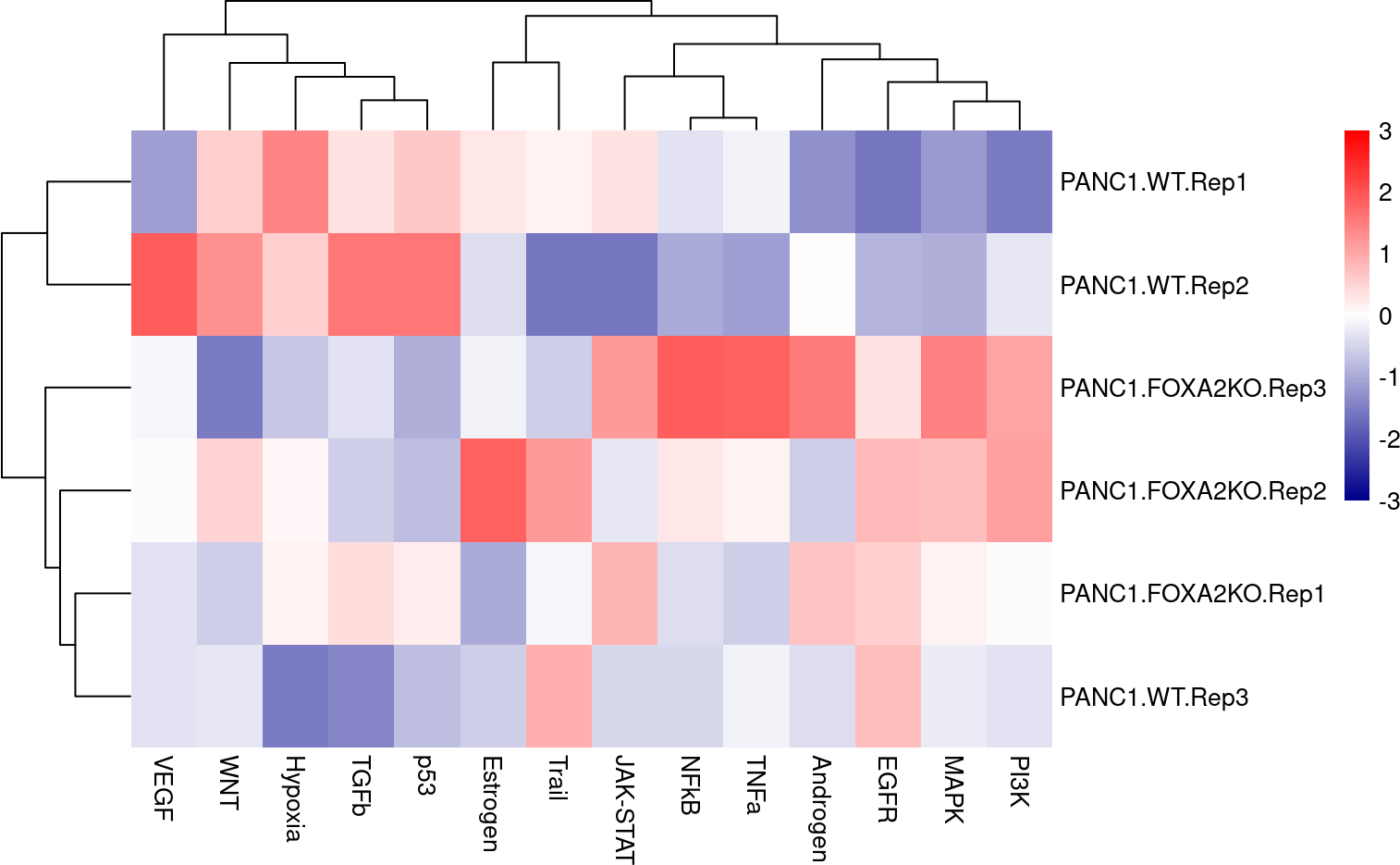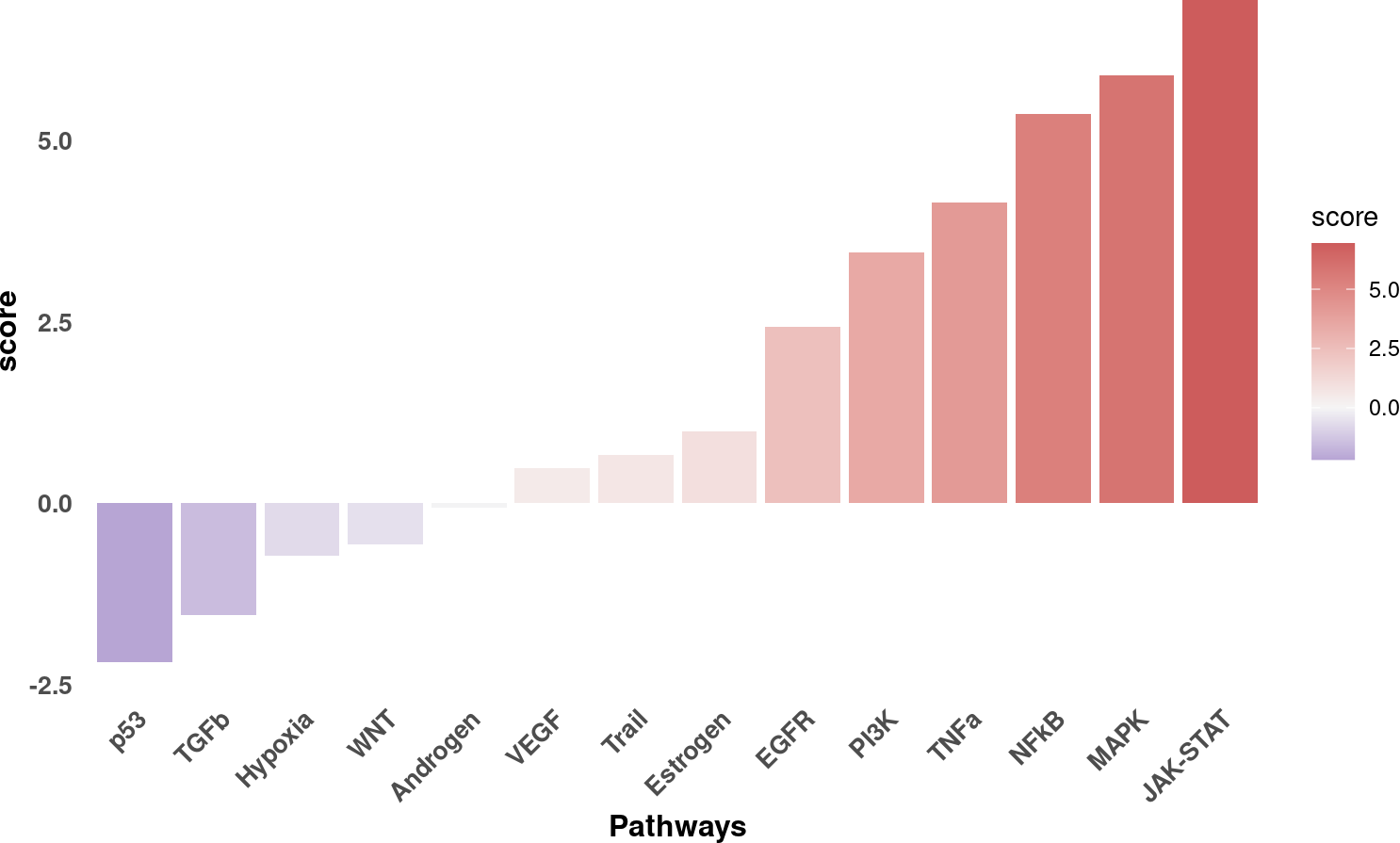Bulk RNA-seq yield many molecular readouts that are hard to interpret by themselves. One way of summarizing this information is by inferring pathway activities from prior knowledge.

In this notebook we showcase how to use `decoupleR` for pathway activity inference with a bulk RNA-seq data-set where the transcription factor FOXA2 was knocked out in pancreatic cancer cell lines.

The data consists of 3 Wild Type (WT) samples and 3 Knock Outs (KO). They are freely available in GEO.

First, we need to load the relevant packages:

``````## We load the required packages
library(decoupleR)
library(dplyr)
library(tibble)
library(tidyr)
library(ggplot2)
library(pheatmap)
library(ggrepel)``````

Here we used an already processed bulk RNA-seq data-set. We provide the normalized log-transformed counts, the experimental design meta-data and the Differential Expressed Genes (DEGs) obtained using `limma`. We can open the data like this:

``````inputs_dir <- system.file("extdata", package = "decoupleR")

From `data` we can extract the mentioned information. Here we see the normalized log-transformed counts:

``````# Remove NAs and set row names
counts <- data\$counts %>%
dplyr::mutate_if(~ any(is.na(.x)), ~ if_else(is.na(.x),0,.x)) %>%
column_to_rownames(var = "gene") %>%
as.matrix()
#>          PANC1.WT.Rep1 PANC1.WT.Rep2 PANC1.WT.Rep3 PANC1.FOXA2KO.Rep1 PANC1.FOXA2KO.Rep2 PANC1.FOXA2KO.Rep3
#> NOC2L        10.052588     11.949123     12.057774          12.312291          12.139918          11.494205
#> PLEKHN1       7.535115      8.125993      8.714880           8.048196           8.290154           8.621239
#> PERM1         6.281242      6.424582      6.589668           6.293285           6.486136           6.775344
#> ISG15        10.938252     11.469081     11.425415          11.549986          11.371464          11.178157
#> AGRN          6.956335      7.196108      7.522550           7.061549           7.485534           7.071555
#> C1orf159      9.546224      9.788721      9.794589           9.850830           9.988069           9.965357``````

The design meta-data:

``````design <- data\$design
design
#> # A tibble: 6 × 2
#>   sample             condition
#>   <chr>              <chr>
#> 1 PANC1.WT.Rep1      PANC1.WT
#> 2 PANC1.WT.Rep2      PANC1.WT
#> 3 PANC1.WT.Rep3      PANC1.WT
#> 4 PANC1.FOXA2KO.Rep1 PANC1.FOXA2KO
#> 5 PANC1.FOXA2KO.Rep2 PANC1.FOXA2KO
#> 6 PANC1.FOXA2KO.Rep3 PANC1.FOXA2KO``````

And the results of `limma`, of which we are interested in extracting the obtained t-value from the contrast:

``````# Extract t-values per gene
deg <- data\$limma_ttop %>%
select(ID, t) %>%
filter(!is.na(t)) %>%
column_to_rownames(var = "ID") %>%
as.matrix()
#>                  t
#> RHBDL2  -12.810588
#> PLEKHH2 -10.794453
#> HEG1     -9.788112
#> CLU      -9.761618
#> FHL1      8.950191
#> RBP4     -8.529074``````

# 3 PROGENy model

PROGENy is a comprehensive resource containing a curated collection of pathways and their target genes, with weights for each interaction. For this example we will use the human weights (mouse is also available) and we will use the top 100 responsive genes ranked by p-value. We can use `decoupleR` to retrieve it from `OmniPath`:

``````net <- get_progeny(organism = 'human', top = 100)
net
#> # A tibble: 1,400 × 4
#>    source   target  weight  p_value
#>    <chr>    <chr>    <dbl>    <dbl>
#>  1 Androgen TMPRSS2  11.5  2.38e-47
#>  2 Androgen NKX3-1   10.6  2.21e-44
#>  3 Androgen MBOAT2   10.5  4.63e-44
#>  4 Androgen KLK2     10.2  1.94e-40
#>  5 Androgen SARG     11.4  2.79e-40
#>  6 Androgen SLC38A4   7.36 1.25e-39
#>  7 Androgen MTMR9     6.13 2.53e-38
#>  8 Androgen ZBTB16   10.6  1.57e-36
#>  9 Androgen KCNN2     9.47 7.71e-36
#> 10 Androgen OPRK1    -5.63 1.11e-35
#> # … with 1,390 more rows``````

# 4 Activity inference with Weighted Mean

To infer activities we will run the Weighted Mean method (`wmean`). It infers regulator activities by first multiplying each target feature by its associated weight which then are summed to an enrichment score `wmean`. Furthermore, permutations of random target features can be performed to obtain a null distribution that can be used to compute a z-score `norm_wmean`, or a corrected estimate `corr_wmean` by multiplying `wmean` by the minus log10 of the obtained empirical p-value.

In this example we use `wmean` but we could have used any other. To see what methods are available use `show_methods()`.

To run `decoupleR` methods, we need an input matrix (`mat`), an input prior knowledge network/resource (`net`), and the name of the columns of net that we want to use.

``````# Run wmean
sample_acts <- run_wmean(mat=counts, net=net, .source='source', .target='target',
.mor='weight', times = 100, minsize = 5)
sample_acts
#> # A tibble: 252 × 5
#>    statistic  source   condition            score p_value
#>    <chr>      <chr>    <chr>                <dbl>   <dbl>
#>  1 corr_wmean Androgen PANC1.FOXA2KO.Rep1  4.24     0.26
#>  2 corr_wmean Androgen PANC1.FOXA2KO.Rep2  6.07     0.140
#>  3 corr_wmean Androgen PANC1.FOXA2KO.Rep3  2.87     0.4
#>  4 corr_wmean Androgen PANC1.WT.Rep1       7.10     0.100
#>  5 corr_wmean Androgen PANC1.WT.Rep2       3.93     0.28
#>  6 corr_wmean Androgen PANC1.WT.Rep3       6.59     0.120
#>  7 corr_wmean EGFR     PANC1.FOXA2KO.Rep1  0.0188   0.82
#>  8 corr_wmean EGFR     PANC1.FOXA2KO.Rep2  0.0224   0.8
#>  9 corr_wmean EGFR     PANC1.FOXA2KO.Rep3  0.0372   0.66
#> 10 corr_wmean EGFR     PANC1.WT.Rep1      -0.0520   0.28
#> # … with 242 more rows``````

# 5 Visualization

From the obtained results, we will select the `norm_wmean` activities and we will observe the obtained activities per sample in a heat-map:

``````# Transform to wide matrix
sample_acts_mat <- sample_acts %>%
filter(statistic == 'norm_wmean') %>%
pivot_wider(id_cols = 'condition', names_from = 'source',
values_from = 'score') %>%
column_to_rownames('condition') %>%
as.matrix()

# Scale per sample
sample_acts_mat <- scale(sample_acts_mat)

# Choose color palette
palette_length = 100
my_color = colorRampPalette(c("Darkblue", "white","red"))(palette_length)

my_breaks <- c(seq(-3, 0, length.out=ceiling(palette_length/2) + 1),
seq(0.05, 3, length.out=floor(palette_length/2)))

# Plot
pheatmap(sample_acts_mat, border_color = NA, color=my_color, breaks = my_breaks) ``````We can observe that WT samples have higher activities for p53 and TGFb than KO. On the other hand, KO show higher activities for MAPK and PI3K.

We can also infer pathway activities from the t-values of the DEGs between KO and WT:

``````# Run wmean
contrast_acts <- run_wmean(mat=deg, net=net, .source='source', .target='target',
.mor='weight', times = 100, minsize = 5)
contrast_acts
#> # A tibble: 42 × 5
#>    statistic  source   condition    score p_value
#>    <chr>      <chr>    <chr>        <dbl>   <dbl>
#>  1 corr_wmean Androgen t         -0.00231  0.0200
#>  2 corr_wmean EGFR     t          0.533    0.1
#>  3 corr_wmean Estrogen t          0.0550   0.48
#>  4 corr_wmean Hypoxia  t         -0.00295  0.94
#>  5 corr_wmean JAK-STAT t          1.90     0.02
#>  6 corr_wmean MAPK     t          1.99     0.02
#>  7 corr_wmean NFkB     t          2.04     0.02
#>  8 corr_wmean PI3K     t          0.962    0.02
#>  9 corr_wmean TGFb     t         -0.217    0.2
#> 10 corr_wmean TNFa     t          1.58     0.02
#> # … with 32 more rows``````

We select the `norm_wmean` activities and then we show the changes in activity between KO and WT:

``````# Filter norm_wmean
f_contrast_acts <- contrast_acts %>%
filter(statistic == 'norm_wmean')

# Plot
ggplot(f_contrast_acts, aes(x = reorder(source, score), y = score)) +
geom_bar(aes(fill = score), stat = "identity") +
scale_fill_gradient2(low = "darkblue", high = "indianred",
mid = "whitesmoke", midpoint = 0) +
theme_minimal() +
theme(axis.title = element_text(face = "bold", size = 12),
axis.text.x =
element_text(angle = 45, hjust = 1, size =10, face= "bold"),
axis.text.y = element_text(size =10, face= "bold"),
panel.grid.major = element_blank(),
panel.grid.minor = element_blank()) +
xlab("Pathways")``````As observed before, the pathways p53 and TGFb are deactivated in KO when compared to WT, while JAK-STAT and others seem to be activated.

We can further visualize the most responsive genes in each pathway along their t-values to interpret the results. For example, let’s see the genes that are belong to the MAPK pathway:

``````pathway <- 'MAPK'

df <- net %>%
filter(source == pathway) %>%
arrange(target) %>%
mutate(ID = target, color = "3") %>%
column_to_rownames('target')
inter <- sort(intersect(rownames(deg),rownames(df)))
df <- df[inter, ]
df['t_value'] <- deg[inter, ]
df <- df %>%
mutate(color = if_else(weight > 0 & t_value > 0, '1', color)) %>%
mutate(color = if_else(weight > 0 & t_value < 0, '2', color)) %>%
mutate(color = if_else(weight < 0 & t_value > 0, '2', color)) %>%
mutate(color = if_else(weight < 0 & t_value < 0, '1', color))

ggplot(df, aes(x = weight, y = t_value, color = color)) + geom_point() +
scale_colour_manual(values = c("red","royalblue3","grey")) +
geom_label_repel(aes(label = ID)) +
theme_minimal() +
theme(legend.position = "none") +
geom_vline(xintercept = 0, linetype = 'dotted') +
geom_hline(yintercept = 0, linetype = 'dotted') +
ggtitle(pathway)
#> Warning: ggrepel: 66 unlabeled data points (too many overlaps). Consider increasing max.overlaps``````